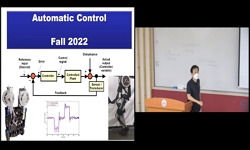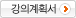### 주메뉴

### 자동제어

• 세종대학교
• 곽관웅• 주제분류
공학 >기계ㆍ금속 >기계공학
• 강의학기
2022년 2학기
• 조회수
9,462
•
강의계획서This course introduces the basic priciples of feedback control of dynamic system, related system analysis technics, and the design of the controller satisfying the given dynamic specifications. To achieve the course goals, system odeling technics descrbing the dynamic systems into mathematical differential equations, technics (root locus, Nyquist) analyzing the dynamic characteristics (stability, tranqusient response) in both time and frequency domain ill be studied. Also, PID and lead-lag controller design method that makes the system dynamic characteristics satisfy the required specifications will be investigated.
Introduction to Feedback Control#### 차시별 강의1.Introduction to Feedback ControlThe Laplace Transform2.The Laplace Transform (Inverse Laplace Transform, Partial Fraction Expansion)Solving linear time-invariant differential equations using L.T.3.Transfer Functions, Impulse responseBlock diagrams, block diagram reduction4.Block diagram reduction, Signal flow graph (1)Block diagram reduction, Signal flow graph (2)Signal flow graph, Mason’s rule (1)Signal flow graph, Mason’s rule (2)5.State space representationConverting s.s. to a T.F. and TF to SS6.Linearization-1st order system, 2nd order system (1)Linearization-1st order system, 2nd order system (2)Linearization-1st order system, 2nd order system (3)7.2nd order system, Higher order systems, Stability analysisHigher order systems, Stability analysis, Steady-state errors in unity feedback control systems8.Static error constant, Steady-state error for nonunity feedbackRoot-Locus Analysis (Root-Locus plots, pole-zero cancellation)9.Root-Locus Analysis (Nonminimum phase system, positive feedback system, conditionally stable system) (1)Root-Locus Analysis (Nonminimum phase system, positive feedback system, conditionally stable system) (2)Control Systems Design by the Root-Locus Method (Lead compensation)10.Control Systems Design by the Root-Locus Method (Lag compensation, Lead-Lag compensation)Control Systems Design by the Root-Locus Method (Lead-Lag compensation, paralle compensation, velocity feedback)11.Frequency Response Analysis (Bode plots of first-order factors)Frequency Response Analysis (Bode plots of first-order & quadratic factors)12.Frequency Response Analysis (system types and log-magnitude curve)Frequency Response Analysis (polar plots)13.Frequency Response Analysis (Nyquist stability criterion)Frequency Response Analysis (Nyquist stability criterion, relative stability)14.Control Systems Design by Frequency Response (Lead compensation, Lag compensation, Lead-Lag compensation)#### 연관 자료#### 사용자 의견

강의 평가를 위해서는 로그인 해주세요.

#### 이용방법

• 동영상 유형 강의 이용시 필요한 프로그램 [바로가기]

※ 강의별로 교수님의 사정에 따라 전체 차시 중 일부 차시만 공개되는 경우가 있으니 양해 부탁드립니다.